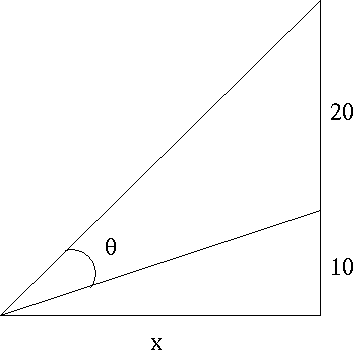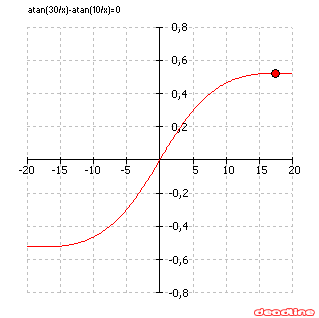# Solve optimization problems: find maximum and minimum

Optimization problem

A movie screen on a wall is 20 feet high and 10 feet above the floor. At what distance x from the front of the room should you position yourself so that the viewing angle of the movie screen is as large as possible ?If x is the distance we want to find, we can conclude that x>0. Let's note α the angle next to θ.

tan(α)=10/x

tan(α+θ)=30/x

α=atan(10/x)

α+θ=atan(30/x)

θ=atan(30/x)-atan(10/x)If you use DeadLine to find extrema of the function f(x)=atan(30/x)-atan(10/x), you will get x=17.32050808 feet, which is the answer to the problem.

Another optimization problem:
Find the dimensions of the rectangle of largest area which can be inscribed in the closed region bounded by the x-axis, y-axis, and graph of f(x)=8-x3. See the solution.

Solve optimization problems, find maximum and minimum of a function using DeadLine.

DeadLine OnLine - free equation solver. Copyright 2003-2007 Ionut Alex. Chitu. | Contact | Sitemap